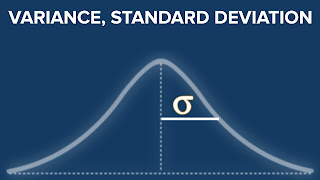## Posts

Showing posts from September, 2020

### Why we do not use standard divination instead to mean absolute error in Regression Models?As usual, My colleague "Jose" surprised "Austin Lasseter" (our Instructor) with a good question, and make the class stop for a while. This time Jose made a shock for all of us when he notes that the calculation of the "mean absolute error" is so close to the calculation of the "standard deviation". then he asks "why not using standard deviation?" he means Why we do not use standard divination instead to mean absolute error in Regression Models? Actually, when I focused more, I found something really fantastic, the "mean absolute error" which we study in Data science to make a Regression is the same the "Average Deviation"  or we commonly called it "The mean absolute deviation (MAD)" which we use it to measure the volatility of returns of the portfolio (in investment). And in the investment, we prefer to use the standard deviation rather than "The mean absolute deviation (MAD)", as both measures th